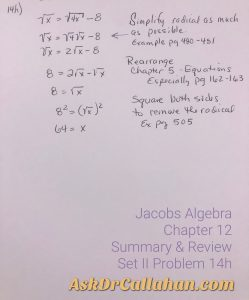Posted on

## Homework Help Complex Problems – Moving Deeper into Concepts

As you move deeper into each textbook (Algebra, Geometry, Calculus, etc), the problems will contain several concepts you’ve learned earlier – all in one problem.  This complexity can make it difficult to find the “how-to” or examples in the book to help us understand how to work it.

Recently, we received this question from our student support page: There doesn’t seem to be any examples or teaching that I can find that helps me solve this problem.

This is a common question – not this particular problem, but in general, as the problems develop into including several simple concepts stacked into compound calculations. However, the explanations are there, they just may be back a few, or several, chapters.

For example, Jacobs Algebra Chapter 12 Summary and Review Problem 14h.Concepts include but are not limited to:

• Chapter 12: Square Roots. Simplify radical as much as possible. Example of this step page 480-481
• Chapter 5: Equations in One Variable. Specifically for this review problem, Equivalent Equations (Lesson 3) page 162-163.
• Chapter 12: Square Roots: Radical Equations. Page 505 has examples of squaring both sides to eliminate the radical.

Math builds on itself. As you learn more and more concepts, the problems reach back and build on calculating and analyzing skills learned earlier in the book or even in an earlier course. These complex problems can be hard to find “how-to” when we just can’t see it! The solutions manual is a good resource to help with steps, but sometimes even with those steps, we need to see where it was explained or taught.

We are here to help! Send us your homework questions and let us help. Filling out this form makes it easy to be sure you’ve told us what we need to know to help you, but you can also send an email to support@askdrcallahan.com.

## Be sure to tell us:

1. the course,
2. the chapter,
3. the lesson,
4. the problem,
5. YOUR issue as best as you can explain it.

We love to help.